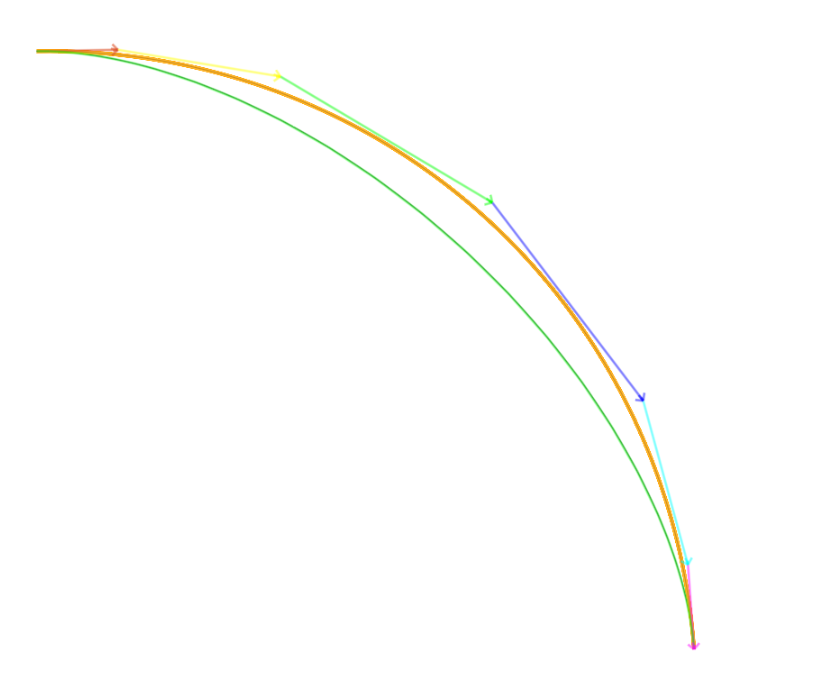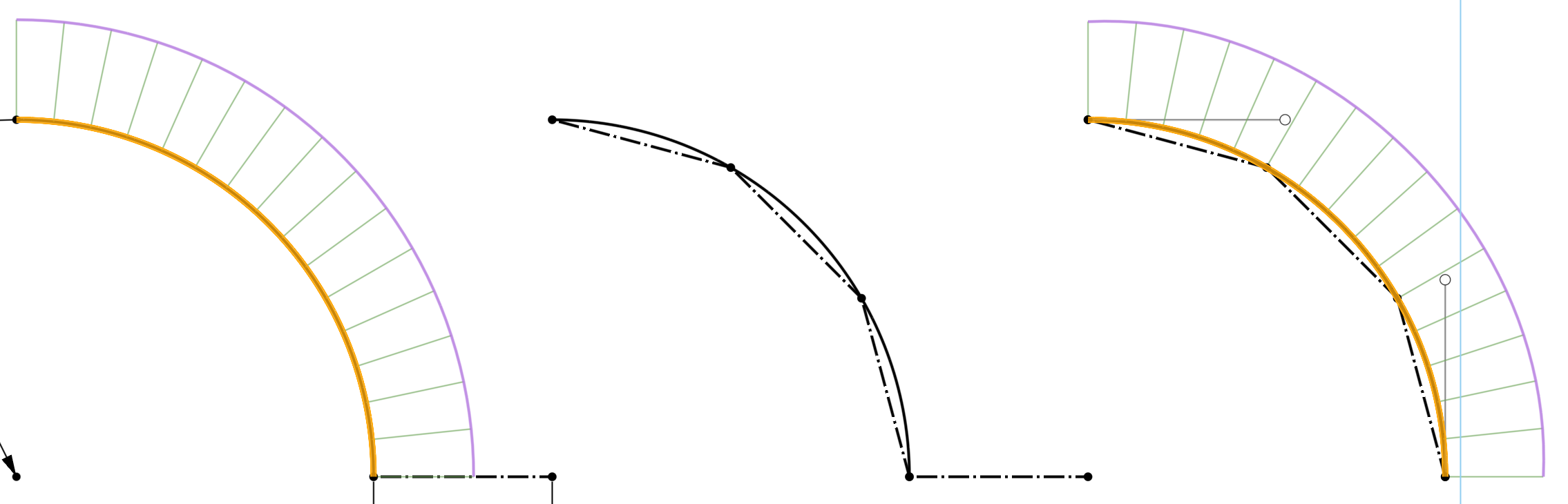Welcome to the Onshape forum! Ask questions and join in the discussions about everything Onshape.

First time visiting? Here are some places to start:
1. Looking for a certain topic? Check out the categories filter or use Search (upper right).
2. Need support? Ask a question to our Community Support category.
3. Please submit support tickets for bugs but you can request improvements in the Product Feedback category.
4. Be respectful, on topic and if you see a problem, Flag it.

If you would like to contact our Community Manager personally, feel free to send a private message or an email.

# set degree of approximate b-spline?

Is there a way to use evApproximateBSplineCurve and get a curve with a set degree other than 3?
Evan Reese / Principal and Industrial Designer with Ovyl
Website: ovyl.io

• @Evan_Reese
Not that I can see so far. As I was saying in another thread, I would really like an option to return a (single span) Bézier, at the potential expense of accuracy.
• @Evan_Reese

You can. There is a feature called unified spline that has the code to raise the degree of the spline. In this feature it is used to combine b spline curves so the degrees need to match. It just adds additional knots at each control point.

For a true b spline curve changing the degree will have a different type of impact if the knots are not padded as done in the unified spline feature.

Here is the unified spline feature

function is spline_IncrementSplineDegree@S1mon
loop through the control points, take ones that do not touch the last one as evApproximateBSplineCurve groups control points and knots to pin those points on the curve.
Have you tried just calling bSplineCurve({ "degree" : size(controlPoints) - 1, "isPeriodic" : false, "controlPoints" : controlPoints });
this will create a bezier curve and the knot array will be created automatically. Onshape uses this in bridging curve. You can find the code in bridgingCurve.fs

create fs

extract points
call bSplineCurve({ "degree" : size(controlPoints) - 1, "isPeriodic" : false, "controlPoints" : controlPoints });
call opCreateBSplineCurve

Here is the result of converting a b spline curve into a bezier curve. the orange is the original b spline curve degree 3, the green spline is the bezier curve. If you want the bezier curve to follow the exact spline path of the original spline, then the control points would need to be moved away or the original spline would have needed to be created as a bezier curve. control points would have been the same, the knots would be different.

if you want to know if it is a bezier curve, check the degree. if its degree is control point count -1 then it might be.• @Jacob_Corder

Thanks!
I had not seen unified spline before. There's a lot to dig through in there. I'm still trying to figure out the goal for unified spline. It seems like a fit spline from Solidworks - something that takes input curves/edges (possibly with gaps) and makes a single spline. I thought that 3DFitSpline did that already. I'm also not seeing very smooth curvatures on the examples. Before Solidworks got decent "style" splines, I would use its fitspline to make C2 transitions from straight lines to arcs, by leaving gaps and tuning the locations.

My goal is definitely to have a rebuilt curve match closely with the input edge/curve (within some tolerance of course).
• @S1mon

its goal is to combine multiple curves into one by approximating the curve. it will not create bezier curves for you though.

If you find a way to calculate a bezier from a non bezier while still keeping the degree under the max, please share it because its likely not possible because one thing you need to keep in mind is that control points are not supposed to touch the spline. they do at start and end because it is padded with degree + 1 of the same knot value.

I believe a bezier exists between controlPoint[n] and controlPoint[n+1]. you could create a bunch of sub beziers from the bsplinecurve and then combine them into one massive b spline curve.  But i am not totally sure this is true.

I have code to combine b splines but cannot share it here. if you get to that point, the b spline curve combining exists in unified spline. the key to making it work was normalizing the knots for each b spline based on the length of each curve. I wish i could tell you more.

• edited February 2022
@Jacob_Corder

In Solidworks, I used to approximate 2D curves with Béziers all the time using some simple geometric techniques. First I would place a series of points equally spaced along the curve (using straight line segments set to be equal). Then I would add a Bézier spline of the minimum needed degree to get a decent match, and then align the spline with the sampled points from the curve. I would also align the end tangents with the curve to be simplified. If I didn't get a good enough match, I would increase the degree (and the number of sampled points). In many cases I would set the lengths of the control polygon sides to all be equal. In some cases I would use C2 constraints on one or both ends, but usually this would over-constrain what I was trying to do.This is a quick example in Onshape. The first step (left) curve is an arc. The second step (middle) is adding the 1/3 and 2/3 points on the arc. The last step (right) is adding a spline (limited to degree 3 in Onshape sketcher) and constraining the two end points, 1/3 and 2/3 points, and the tangent angles. The solver handles the tangent magnitudes. This is not a perfect arc (and mathematically never can be with Béziers) but it's a close approximation with a very simple algorithm.

Although I can easily write the pseudo code for this algorithm, writing the actual code in FeatureScript, especially the 3D constraint solver, is a bit daunting to me.

This technique will not work for any random curve. It's really only useful for stuff with limited changes in curvature and degree.